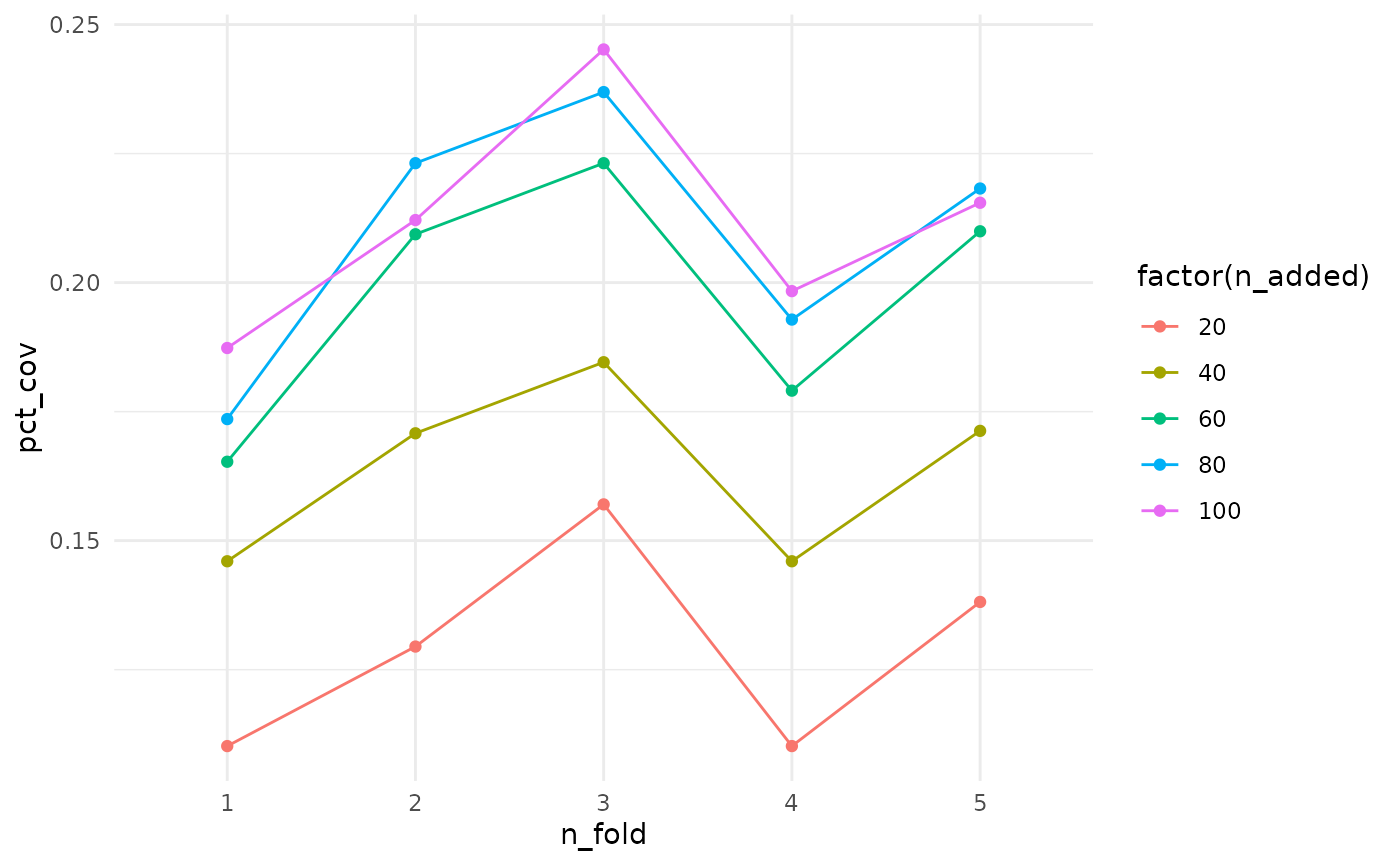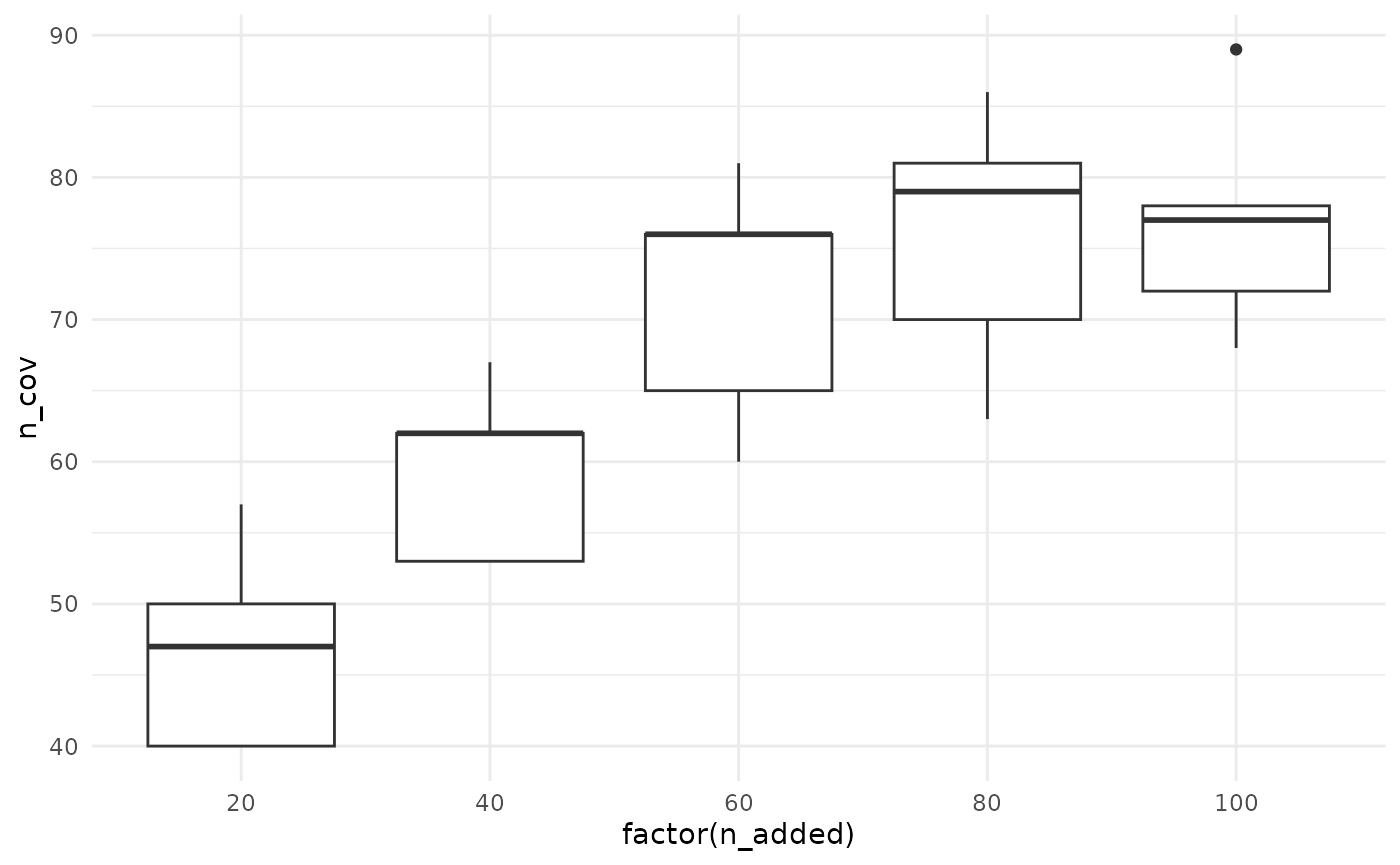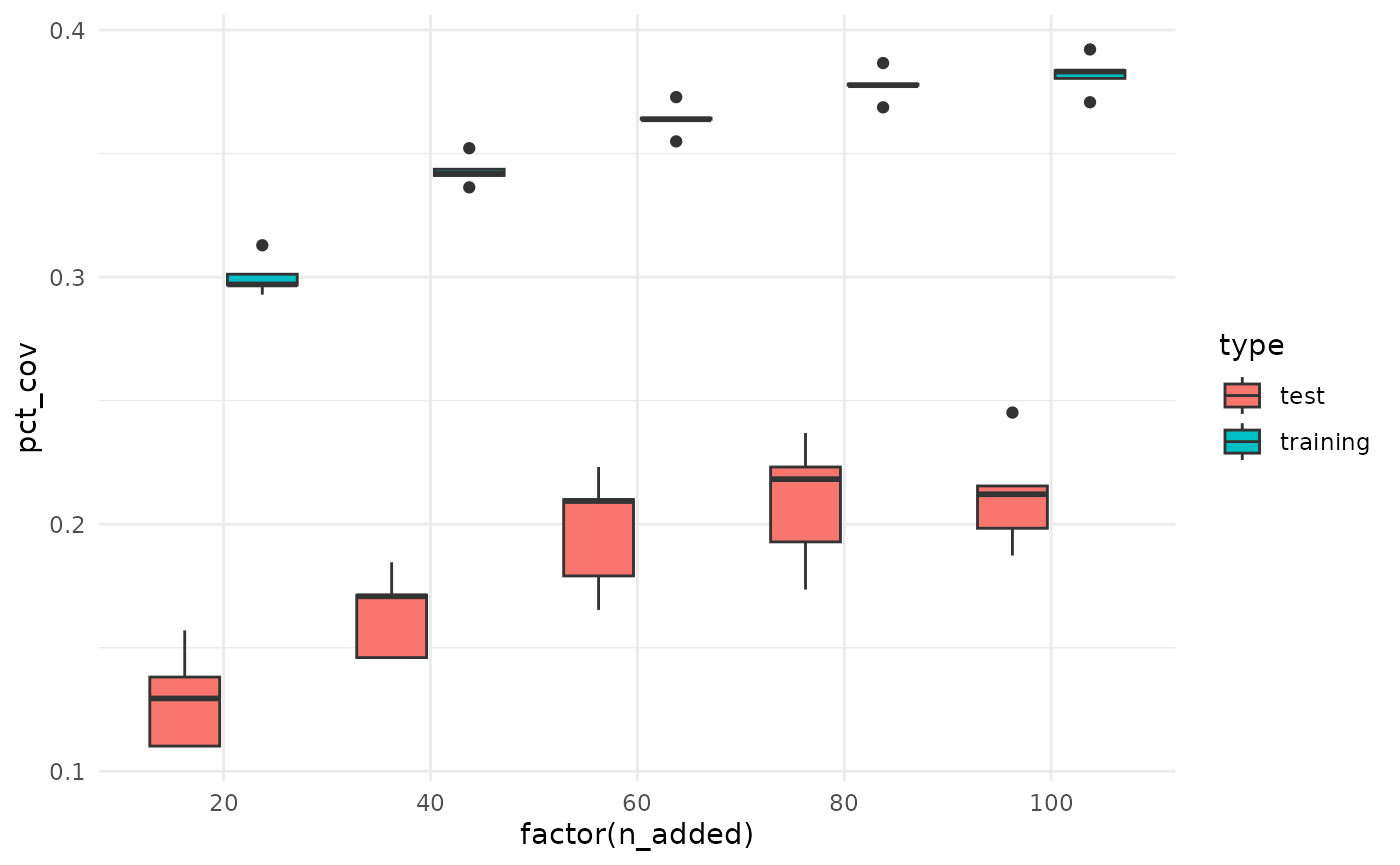This vignette provides a guide to performing cross validation with modelr and maxcovr. Cross validation is a bit of a strange concept in this context - we aren’t trying to make predictions here, but what we are trying to do is appropriately evaluate the performance of maxcovr in a fairer way.

In the future if this is a feature that is under demand, I will incorporate cross validation into maxcovr. In the mean time, here’s a vignette.

# Performing cross validation on max_coverage

We will stick with the previous example using york and york_crime

library(maxcovr)
library(dplyr)
##
## Attaching package: 'dplyr'
## The following objects are masked from 'package:stats':
##
##     filter, lag
## The following objects are masked from 'package:base':
##
##     intersect, setdiff, setequal, union
# subset to be the places with towers built on them.
york_selected <- york %>% filter(grade == "I")

york_unselected <- york %>% filter(grade != "I")

Thanks to the modelr package, it is relatively straightforward to perform cross validation.

# first we partition the data into 5 folds
library(modelr)
library(purrr)
library(tibble)
mc_cv <- modelr::crossv_kfold(york_crime, 5) %>%
# we change the test and train sets from the resample
# to tibbles
mutate(test = map(test,as_tibble),
train = map(train,as_tibble))

This creates a dataframe with test and training sets

mc_cv
## # A tibble: 5 x 3
##   train                 test                .id
##   <list>                <list>              <chr>
## 1 <tibble [1,451 × 12]> <tibble [363 × 12]> 1
## 2 <tibble [1,451 × 12]> <tibble [363 × 12]> 2
## 3 <tibble [1,451 × 12]> <tibble [363 × 12]> 3
## 4 <tibble [1,451 × 12]> <tibble [363 × 12]> 4
## 5 <tibble [1,452 × 12]> <tibble [362 × 12]> 5

We then fit the model on the training set using map_df, from the purrr package.

library(purrr)
# then we fit the model
system.time(
mc_cv_fit <- map_df(mc_cv$train, ~max_coverage(existing_facility = york_selected, proposed_facility = york_unselected, user = ., # training set goes here n_added = 20, distance_cutoff = 100)) ) ## user system elapsed ## 3.536 0.423 3.978 Then we can use the summary_mc_cv function to extract out the summaries from each fold. This summary takes the facilities placed using the training set of users, and then takes the test set of users and counts what percent of these are being covered by the training model. summarised_cv <- summary_mc_cv(mc_cv_fit, mc_cv) summarised_cv %>% knitr::kable() n_added n_fold distance_within n_cov pct_cov n_not_cov pct_not_cov dist_avg dist_sd 20 1 100 58 0.1597796 305 0.8402204 1065.714 1382.361 20 2 100 45 0.1239669 318 0.8760331 1175.605 1604.221 20 3 100 37 0.1019284 326 0.8980716 1325.302 1703.502 20 4 100 53 0.1460055 310 0.8539945 1024.408 1341.573 20 5 100 49 0.1353591 313 0.8646409 1104.269 1310.461 Eyeballing the values, it looks like the pct coverage stays around 10%, but we can plot it to get a better idea. We can overlay the coverage obtained using the full dataset to get an idea of how we are performing. library(ggplot2) summarised_cv %>% ggplot(aes(x = n_fold, y = pct_cov)) + geom_point() + geom_line(group = 1) + theme_minimal()Here we see that the pct_coverage doesn’t seem to change much across the folds. Coming up next, we will explore how to perform cross validation as we increase the number of facilities added. Ideally, there should be a way to do this using purrr, so we don’t have to fic 5 separate models, but perhaps this will change when we enable n_added to take a vector of values. # then we fit the model system.time( mc_cv_fit_n20 <- map_df(mc_cv$train,
~max_coverage(existing_facility = york_selected,
proposed_facility = york_unselected,
user = ., # training set goes here
distance_cutoff = 100))
)
##    user  system elapsed
##   3.329   0.412   3.745
system.time(
mc_cv_fit_n40 <- map_df(mc_cv$train, ~max_coverage(existing_facility = york_selected, proposed_facility = york_unselected, user = ., # training set goes here n_added = 40, distance_cutoff = 100)) ) ## user system elapsed ## 3.400 0.332 3.739 system.time( mc_cv_fit_n60 <- map_df(mc_cv$train,
~max_coverage(existing_facility = york_selected,
proposed_facility = york_unselected,
user = ., # training set goes here
distance_cutoff = 100))
)
##    user  system elapsed
##   3.737   0.344   4.089
system.time(
mc_cv_fit_n80 <- map_df(mc_cv$train, ~max_coverage(existing_facility = york_selected, proposed_facility = york_unselected, user = ., # training set goes here n_added = 80, distance_cutoff = 100)) ) ## user system elapsed ## 3.665 0.453 4.124 system.time( mc_cv_fit_n100 <- map_df(mc_cv$train,
~max_coverage(existing_facility = york_selected,
proposed_facility = york_unselected,
user = ., # training set goes here
distance_cutoff = 100))
)
##    user  system elapsed
##  31.324   0.593  32.095
summarised_cv_n20 <- summary_mc_cv(mc_cv_fit_n20, mc_cv)
summarised_cv_n40 <- summary_mc_cv(mc_cv_fit_n40, mc_cv)
summarised_cv_n60 <- summary_mc_cv(mc_cv_fit_n60, mc_cv)
summarised_cv_n80 <- summary_mc_cv(mc_cv_fit_n80, mc_cv)
summarised_cv_n100 <- summary_mc_cv(mc_cv_fit_n100, mc_cv)

bound_testing_summaries <- bind_rows(summarised_cv_n20,
summarised_cv_n40,
summarised_cv_n60,
summarised_cv_n80,
summarised_cv_n100) %>%
mutate(type = "test")

It looks like the more facilities we add, the better the coverage…mostly.

bound_training_summaries <- bind_rows(mc_cv_fit_n20$model_coverage, mc_cv_fit_n40$model_coverage,
mc_cv_fit_n60$model_coverage, mc_cv_fit_n80$model_coverage,
mc_cv_fit_n100\$model_coverage) %>%
mutate(type = "training")

bound_all_summaries <- bind_rows(bound_testing_summaries,
bound_training_summaries)
ggplot(bound_testing_summaries,
aes(x = n_fold,
y = pct_cov,
geom_point() +
geom_line() +
theme_minimal()Let’s look at this another way, with boxplots for the number of facilities added.

ggplot(bound_testing_summaries,
y = n_cov)) +
geom_boxplot() +
theme_minimal()We can also compare the % coverage for the test and training datasets

bound_all_summaries %>%
theme_minimal()